Next: Huygens' principle Up: Wave Optics Previous: Wave Optics

## Introduction

Geometric optics is an incredibly successful theory. Probably its most important application is in describing and explaining the operation of commonly occurring optical instruments: e.g., the camera, the telescope, and the microscope. Although geometric optics does not make any explicit assumption about the nature of light, it tends to suggest that light consists of a stream of massless particles. This is certainly what scientists, including, most notably, Isaac Newton, generally assumed up until about the year 1800.

Let us examine how the particle theory of light accounts for the three basic laws of geometric optics:

1. The law of geometric propagation: This is easy. Massless particles obviously move in straight-lines in free space.
2. The law of reflection: This is also fairly easy. We merely have to assume that light particles bounce elastically (i.e., without energy loss) off reflecting surfaces.
3. The law of refraction: This is the tricky one. Let us assume that the speed of light particles propagating through a transparent dielectric medium is proportional to the index of refraction,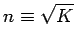. Let us further assume that at a general interface between two different dielectric media, light particles crossing the interface conserve momentum in the plane parallel to the interface. In general, this implies that the particle momenta normal to the interface are not conserved: i.e., the interface exerts a normal reaction force on crossing particles, but no parallel force. From Fig. 83, parallel momentum conservation for light particles crossing the interface yields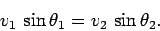(368)

However, by assumption,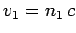and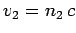, so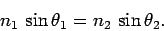(369)

This highly contrived (and incorrect) derivation of the law of refraction was first proposed by Descartes in 1637. Note that it depends crucially on the (incorrect) assumption that light travels faster in dense media (e.g., glass) than in rarefied media (e.g., water). This assumption appears very strange to us nowadays, but it seemed eminently reasonable to scientists in the 17th and 18th centuries. After all, they knew that sound travels faster in dense media (e.g., water) than in rarefied media (e.g., air).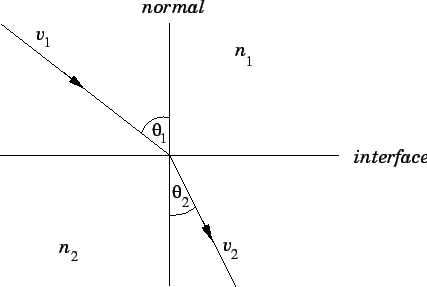The wave theory of light, which became established in the first half of the 19th century, initially encountered tremendous resistance. Let us briefly examine the reasons why scientists in the early 1800s refused to think of light as a wave phenomenon? Firstly, the particle theory of light was intimately associated with Isaac Newton, so any attack on this theory was considered to be a slight to his memory. Secondly, all of the waves that scientists were familiar with at that time manifestly did not travel in straight-lines. For instance, water waves are diffracted as they pass through the narrow mouth of a harbour, as shown in Fig. 84. In other words, the rays'' associated with such waves are bent as they traverse the harbour mouth. Scientists thought that if light were a wave phenomenon then it would also not travel in straight-lines: i.e., it would not cast straight, sharp shadows, any more than water waves cast straight, sharp shadows.'' Unfortunately, they did not appreciate that if the wavelength of light is much shorter than that of water waves then light can be a wave phenomenon and still propagate in a largely geometric manner.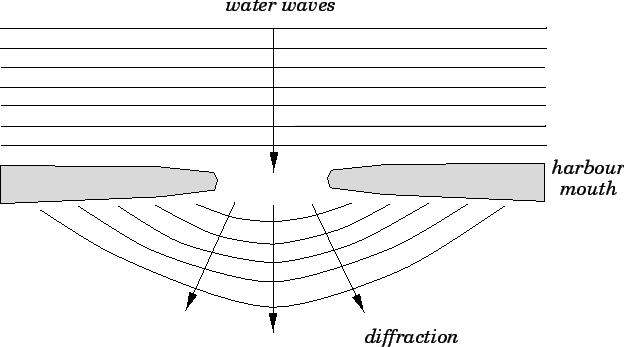Next: Huygens' principle Up: Wave Optics Previous: Wave Optics
Richard Fitzpatrick 2007-07-14